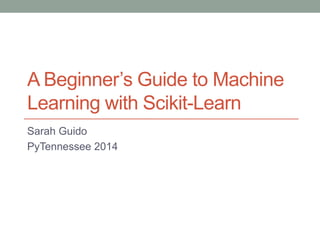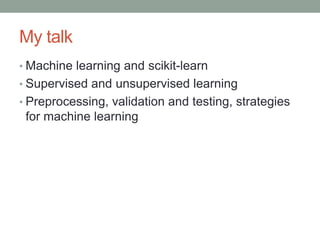Diese Präsentation wurde erfolgreich gemeldet.

# A Beginner's Guide to Machine Learning with Scikit-Learn

Anzeige
Anzeige
Anzeige
Anzeige
Anzeige
Anzeige
Anzeige
Anzeige
Anzeige
Anzeige
Anzeige×

1 von 32 Anzeige

# A Beginner's Guide to Machine Learning with Scikit-Learn

Given at the PyData NYC 2013 conference (http://vimeo.com/79517341), and will be given at PyTennessee 2014.

Scikit-learn is one of the most well-known machine learning Python modules in existence. But how does it work, and what, for that matter, is machine learning? For those with programming experience but who are new to machine learning, this talk gives a beginner-level overview of how machine learning can be useful, important machine learning concepts, and how to implement them with scikit-learn. We’ll use real world data to look at supervised and unsupervised machine learning algorithms and why scikit-learn is useful for performing these tasks.

Given at the PyData NYC 2013 conference (http://vimeo.com/79517341), and will be given at PyTennessee 2014.

Scikit-learn is one of the most well-known machine learning Python modules in existence. But how does it work, and what, for that matter, is machine learning? For those with programming experience but who are new to machine learning, this talk gives a beginner-level overview of how machine learning can be useful, important machine learning concepts, and how to implement them with scikit-learn. We’ll use real world data to look at supervised and unsupervised machine learning algorithms and why scikit-learn is useful for performing these tasks.

Anzeige
Anzeige

### A Beginner's Guide to Machine Learning with Scikit-Learn

1. 1. A Beginner’s Guide to Machine Learning with Scikit-Learn Sarah Guido PyTennessee 2014
2. 2. All about me • Grad student at the University of Michigan • Data analyst for HathiTrust • Organizer of Ann Arbor PyLadies chapter
3. 3. My talk • Machine learning and scikit-learn • Supervised and unsupervised learning • Preprocessing, validation and testing, strategies for machine learning
4. 4. What is machine learning? • Application of algorithms that learn from examples • Representation and generalization
5. 5. Why should we care? • Useful in every day life • Email spam, handwriting analysis, stock market analysis, Netflix • Especially useful in data analysis • Feature extraction, linear regression, classification, clustering
6. 6. Machine Learning Vocab • Instance • Feature • Class • Categorical • Nominal • Ordinal • Continuous
7. 7. Machine Learning Vocab Feature Class Instance
8. 8. Scikit-Learn • Machine learning module • Open-source • Built-in datasets • Good resources for learning
9. 9. Scikit-Learn • Model = EstimatorObject() • Model.fit(dataset.data, dataset.target) • dataset.data = dataset • dataset.target = labels • Model.predict(dataset.data)
10. 10. Scikit-Learn • Supervised • Unsupervised • Semi-supervised • Reinforcement learning • Neural networks • …and many more!
11. 11. Supervised learning • Labeled data • You know what you’re looking for • Classification: predict categorical labels • Regression: predict continuous target variables
12. 12. Classification • Categorical variables • Relationship between instance and feature • Classification algorithms == classifiers
13. 13. Classification • Naïve Bayes classifier • Features are independent • Fast performance • Decent classifier
14. 14. Classification • Car evaluation dataset-UCI • Features: buying price, the maintenance price, the number of doors, the number of seats, the size of the trunk, and the safety ranking • Labels: unacceptable, acceptable, good, or very good
15. 15. Classification
16. 16. Classification
17. 17. Classification
18. 18. Unsupervised algorithms • Unlabeled data • You might have no idea what you’re looking for • Clustering: splitting observations into groups • Dimensionality reduction: flatten data to fewer dimensions
19. 19. Clustering • Exploring the data • Similar objects in the same group • Distance between data points
20. 20. Clustering • K-means clustering • Three steps • Chooses initial cluster centers • Assigns data instance to cluster • Recalculates cluster center • Efficient
21. 21. Clustering
22. 22. Clustering
23. 23. Clustering
24. 24. Data preprocessing • Encoding categorical features
25. 25. Data preprocessing
26. 26. Data preprocessing
27. 27. Data preprocessing • Split the dataset into training and test data
28. 28. Validation and testing • Model evaluation • Cross-validation
29. 29. Good strategies • Avoid overfitting • Use lots of data • Intuition fails in high dimensions
30. 30. My materials • Scikit-learn.org documentation and tutorials • Machine learning class at U of M • Scikit-learn talks
31. 31. Resources • Scikit-learn documentation and tutorials • scikit-learn.org/stable/documentation.html • Other resources • http://archive.ics.uci.edu/ml/datasets.html • Mldata.org • Videos • Scikit-learn tutorial: http://vimeo.com/53062607 • Intro to scikit-learn: http://vimeo.com/72859487
32. 32. Contact me! • @sarah_guido • Linkedin.com/sarahguido • github.com/sarguido

### Hinweis der Redaktion

• Pictures of both
• Snapshot of dataset
• Better explanation of functions
• Better explanation of functions